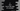# C program to find the first and the last digits of a number## How to find the first and the last digits of a number in C:

In this post, we will learn how to find the first and the last digits of a number in C programming language. The program will take the number as input from the user and print the first and the last digits of the number.

For example, if the number is 1234, the first digit is 1 and the last digit is 4.

### Algorithm to find the first and the last digits of a number:

To find the first and the last digits of a number, we have to use modulo and division. To find the last digit, we can use %10, it will give us the last digit. To find the first digit, we need to keep dividing the number by 10 until we get a single digit.

For example, if the number is 1234, we can use 1234%10 to get 4.

To get the first digit, we will keep dividing the number by 10:

``````1234/10 = 123
123/10 = 12
12/10 = 1``````

We will use this algorithm to find the first and the last digits of a given number.

Let’s write down the program.

### Method 1: C program with loop:

Below is the complete C program:

``````#include <stdio.h>

int getFirstDigit(int no)
{
while (no > 9)
{
no /= 10;
}

return no;
}

int getLastDigit(int no)
{
return no % 10;
}

int main()
{
int n;
printf("Enter the number: ");
scanf("%d", &n);

printf("First digit: %d, Last digit: %d\n", getFirstDigit(n), getLastDigit(n));
return 0;
}``````

In this program,

• getFirstDigit method is used to get the first digit of a number. It takes a number as the parameter and returns the first digit of that number.

• It keeps dividing the number by 10 until a single digit number is received. The single digit is returned, which is the first digit.
• getLastDigit method is used to get the last digit of a number. It also takes a number as the parameter and returns the last digit.

• It uses modulo operator to find the last digit. %10 is used to get the last digit.

If you run this program, it will print output as like below:

``````Enter the number: 1234
First digit: 1, Last digit: 4

Enter the number: 34590
First digit: 3, Last digit: 0``````

### Method 2: C program with log and math:

If we get the total number of digits - 1, it will be easy to find the first digit of a number. For example, for the number 1234, its total digit is 4. If we divide 1234 by 10^3, we will find 1, which is the first digit of that number.

We can use log10 to find the total number of digits - 1 of a number. The value of `(int)log10(number)` returns that.

Let’s write down the program in C;

``````#include <stdio.h>
#include <math.h>

int getFirstDigit(int no)
{
int count = (int)log10(no);

int first = (int)(no / pow(10, count));

return first;
}

int getLastDigit(int no)
{
return no % 10;
}

int main()
{
int n;
printf("Enter the number: ");
scanf("%d", &n);

printf("First digit: %d, Last digit: %d\n", getFirstDigit(n), getLastDigit(n));
return 0;
}``````

We changed the getFirstDigit method to use log and pow. If you run this program, it will print similar output.

This program will be faster than the previous program as we don’t have to use a loop.

### Conclusion:

We learned two different ways to find the first and the last digits of a number in C. You can use any of the above two methods. The second approach is faster than the first approach and for a large number, it will perform better.